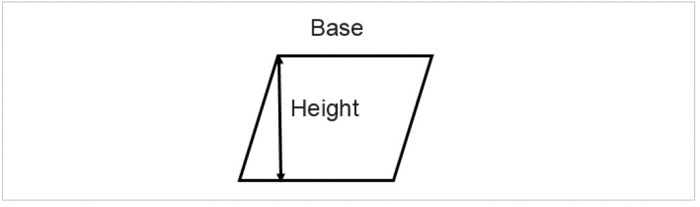# How To Find The Area of a Parallelogram in Golang?

In this tutorial, we are going to see the Golang program to find the Area of a Parallelogram. The area is the total space covered by any closed figure.## Formula

Area of the Parallelogram - base * height
b - length of the base of a Parallelogram
h - length of the height of a Parallelogram


For example, the length of the base of a Parallelogram is 6 cm and the length of the height of the parallelogram is 4 cm so the Area of a Parallelogram is −

b = 6 cm
h = 4 cm
Area = base * height
= 6 * 4
= 24 cm^2


## Finding the Area of a Parallelogram within the function

### Algorithm

Step 1 − Declaring the variables for the length of the base, length of height, and the area of the float64 data type.

Step 2 − Initialize the variable.

Step 3 − Find the Area using the above formula within the function.

Step 4 − Printing the result.

Time Complexity:
O(1)

Space Complexity:
O(1)


## Example 1

In this example, we are going to find the Area of a Parallelogram within the function.

package main
// fmt package provides the function to print anything
import (
"fmt"
)
func main() {
// declaring the floating variables using the var keyword for
// storing the length of the base of the Parallelogram,
// length of the height of the Parallelogram and also
// a variable area to store Area
var base, height, Area float64
fmt.Println("Program to find the Area of a Parallelogram.")

// initializing the length of the base of a Parallelogram
base = 6

// initializing the length of the height of a Parallelogram
height = 4

// finding the Area of a Parallelogram
Area = height * base

// printing the result
fmt.Println("The Area of a Parallelogram with base =", base,"and height =", height, "is", Area, "cm * cm.")
fmt.Println("(Finding the Area of a Parallelogram within the function)")
}


## Output

Program to find the Area of a Parallelogram.
The Area of a Parallelogram with base = 6 and height = 4 is 24 cm * cm.
(Finding the Area of a Parallelogram within the function)


## Finding the Area of a Parallelogram in the separate function

### Algorithm

Step 1 − Declaring the variables for the length of the base, length of height, and the area of the float64 data type.

Step 2 − Initialize the variable.

Step 3 − Calling the function with the length of the base of the Parallelogram and the length of the height of the Parallelogram, and storing the Area the function is returning.

Step 4 − Printing the result.

## Example 2

In this example, we are going to find the Area of a Parallelogram by defining the separate function to find the Area.

package main
// fmt package provides the function to print anything
import (
"fmt"
)
// in this line we have declared the function that have float64
// type parameter and float64 type returntype
func areaOfParallelogram(base, height float64) float64 {
// returning the area by applying the formula
return base * height
}
func main() {
// declaring the floating variables using the var keyword for
// storing the length of the base of the Parallelogram,
// length of the height of the Parallelogram and also
// a variable area to store Area
var base, height, Area float64
fmt.Println("Program to find the Area of a Parallelogram.")

// initializing the length of the base of a Parallelogram
base = 6

// initializing the length of the height of a Parallelogram
height = 4

// finding the Area of a Parallelogram by calling the
// function with the respective parameters
Area = areaOfParallelogram(base, height)

// printing the result
fmt.Println("The Area of a Parallelogram with base =", base,"and height =", height, "is", Area, "cm * cm.")
fmt.Println("(Finding the Area of a Parallelogram in the separate function)")
}


## Output

Program to find the Area of a Parallelogram.
The Area of a Parallelogram with base = 6 and height = 4 is 24 cm * cm.
(Finding the Area of a Parallelogram in the separate function)


## Conclusion

These are the two ways to find the Area of a Parallelogram in Golang. The second way is much better in terms of modularity and code reusability as we can call that function anywhere in the project. To learn more about go you can explore these tutorials.